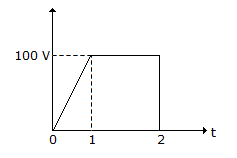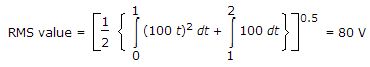# Electronics and Communication Engineering - Networks Analysis and Synthesis - Discussion

2.

The rms value of wave in figure isExplanation:Harman said: (May 28, 2014) Why the power is 0.5?

 Abhishek Das said: (Jul 23, 2014) To obtain root over the power is 1/2 = 0.5.

 Garvit Verma said: (Aug 14, 2014) Is this the formula for rms value of a wave?

 Tej said: (Oct 15, 2014) No rms formula is v^2/sqrt2.

 Lakshmi said: (Dec 6, 2014) I did not under stand you are explanation can you give me more explanation about rms value.

 Kanjanadevi Ramasamy said: (Dec 12, 2014) Can anyone please explain this one elaborately?

 Naresh said: (Dec 26, 2014) In the above formula, integration 1 to 2 it is 100^2.

 Vishal said: (Jan 12, 2015) Yes it should be 100^2 for 1 to 2 integration.

 Midhuna said: (Feb 2, 2015) This formula was confused. Please explain.

 Sunilkumar.Dm said: (Feb 18, 2015) RMS value = sqrt MSV.

 Shantha said: (May 29, 2015) I'm not understanding can anyone please explain clearly.

 Sachin Shukla said: (Jun 6, 2015) RMS value means root mean square which by its name only suggests that it is nothing but the square root of mean of squared value of voltage or any quantity to be measured. In this case the function is voltage which can not be represented by a single function. So the interval of investigation should be broken into respective number sub-intervals where the voltage function can be represented by a single algebraic functions. After getting the square of the functions and do the same as explained in first line.

 Bunny said: (Jul 4, 2015) Why is this 100t not 100 in integration 0 to 1?

 Venky said: (Jul 22, 2015) Because from 0 to 1 it is slope function, y=mt use this formula you will y = 100t. m = 100 i.e, slope. m = (y2-y1)/(x2-x1). RMS = (1/2) (Integral (x (t)^2))^(1/2).

 Abi said: (Sep 28, 2015) How come answer 80? I didn't understand.

 Rakesh said: (Dec 24, 2015) Explain the procedure.

 Naresh said: (Jun 23, 2016) Why the slope m=100 it is 100v. How it is, please explain.

 Arvindkannojiya said: (Aug 2, 2016) Yes in 1 to 2 it should be 100^2.

 Arvindkannojiya said: (Aug 2, 2016) Yes in 1 to 2 it should be 100^2.

 Pratibha said: (Nov 16, 2016) Why 0.5? Please, anyone explain.

 Rashid said: (Nov 23, 2016) It is because of the gravity of the earth.

 Sridhar said: (Dec 5, 2016) Please derive that formula.

 Rajani.Nakka said: (Apr 9, 2017) Great explanations, thanks all of you.

 Mukul said: (Aug 16, 2017) From 1 to 2 it should be 100^2. And if we use 100^2 then answer is different. And if we use 100 only still the answer is different.

 Saniya said: (Nov 15, 2017) From interval 1 to 2 it should be 100^2.

 Vijay said: (Feb 7, 2018) RMS= Root of Mean of Square. As per the given formula...from 1to 2 it is 100^2. => root{1/2[10^4 + 10^4x[1/3] ]}. =>root{1/2[10^4x[4/3] ]}. =>root{10^4 x 2/3}. =>100 x root{2/3}. =>81.someting. = 80(approx).

 Danielle Tampis said: (Feb 20, 2018) RMS or root mean square is the SQUARE ROOT of an area of a specific function SQUARED times (1/2) because of the word MEAN or AVERAGE value. In the given curve, it is divided into two parts. 1. From t = 0 to t = 1, the function is V = 100t (as you can see the value of V is 0 at t = 0 and 100 at t = 1 OR just use point slope form). 2. From t = 1 to t = 2, the area is like a square with sides measuring 100 units. Therefore: vrms = √{(1/2)(A1 + A2)}, Where: A1 = Integral (100t)^2 dt, t = 0 to t = 1. A2 = Integral (100)^2 dt, t = 1 to t = 2.

 Lobomo said: (May 11, 2018) Answer shoulld be 81.64.

 G.Thirunavukkarasu said: (Jun 30, 2018) The given signal consists of a ramp signal plus a dc signal. The ramp signal has the RMS value of Vp/√(3). the dc signal has the RMS value of dc value itself. the total RMS value is; √{(100^2)/3 + 100^2}. This results 200/√(3).

 Sanidhya said: (Jul 10, 2018) Actually, in the first integration the ramp signal is taken so it is 100t and in second integration the step signal is taken 100 (magnitude).